output.to from Sideway
Number Theory

Factorization

Pollard's p-1 Method

Draft for Information Only

# Content

``` Pollard's p-1 Method     Characteristic of Pollard's p-1 Method by Smooth Number     Pollard's P-1 Methed by Smooth Number Example 3```

# Pollard's p-1 Method

Pollard's p-1 method is a prime factorization algorithm discovered by John Pollard in 1974. Limited by the algorithm, the Pollard's p-1 method is only work for integers with specific factors.

However, since the composite number n is an unknown, sometimes, the algorithm may return a false response.

#### Characteristic of Pollard's p-1 Method by Smooth Number

The advantage of Pollard's p-1 method by smooth number is the checking of a group of  primes with one computation. Every boundary B represents a group of numbers that can be expressed as  the product of primes less than and equal to number B.

For example, a composite number n less than 10000 should have a prime factor less than √n=100. And primes within 100 are

Primes Integer B-smooth count
2, 3, 5, 7, 11, 13, 17, 19, 23, 29, 31, 37, 41, 43, 47, 53, 59, 61, 67, 71, 73, 79, 83, 89, 97 p   25
2, 3, 5, 17 p-1 2
2, 3, 5, 7, 13, 17, 19, 37, 73, 97 p-1 3 10
2, 3, 5, 7, 11, 13, 17, 19, 31, 37, 41, 61, 73, 97 p-1 5 14
2, 3, 5, 7, 11, 13, 17, 19, 29, 31, 37, 41, 43, 61, 71, 73, 97 p-1 7 17
2, 3, 5, 7, 11, 13, 17, 19, 23, 29, 31, 37, 41, 43, 61, 67, 71, 73, 89, 97 p-1 11 20
2, 3, 5, 7, 11, 13, 17, 19, 23, 29, 31, 37, 41, 43, 53, 61, 67, 71, 73, 79, 89, 97 p-1 13 22
2, 3, 5, 7, 11, 13, 17, 19, 23, 29, 31, 37, 41, 43, 53, 61, 67, 71, 73, 79, 89, 97 p-1 17 22
2, 3, 5, 7, 11, 13, 17, 19, 23, 29, 31, 37, 41, 43, 53, 61, 67, 71, 73, 79, 89, 97 p-1 19 22
2, 3, 5, 7, 11, 13, 17, 19, 23, 29, 31, 37, 41, 43, 47, 53, 61, 67, 71, 73, 79, 89, 97 p-1 23 23
2, 3, 5, 7, 11, 13, 17, 19, 23, 29, 31, 37, 41, 43, 47, 53, 59, 61, 67, 71, 73, 79, 89, 97 p-1 29 24
2, 3, 5, 7, 11, 13, 17, 19, 23, 29, 31, 37, 41, 43, 47, 53, 59, 61, 67, 71, 73, 79, 89, 97 p-1 31 24
2, 3, 5, 7, 11, 13, 17, 19, 23, 29, 31, 37, 41, 43, 47, 53, 59, 61, 67, 71, 73, 79, 89, 97 p-1 37 24
2, 3, 5, 7, 11, 13, 17, 19, 23, 29, 31, 37, 41, 43, 47, 53, 59, 61, 67, 71, 73, 79, 83, 89, 97 p-1 41 25
2, 3, 5, 7, 11, 13, 17, 19, 23, 29, 31, 37, 41, 43, 47, 53, 59, 61, 67, 71, 73, 79, 83, 89, 97 p-1 43 25
2, 3, 5, 7, 11, 13, 17, 19, 23, 29, 31, 37, 41, 43, 47, 53, 59, 61, 67, 71, 73, 79, 83, 89, 97 p-1 47 25

More primes can be included for checking by increasing the smoothness boundary B.

For checking n and ak-1 is divisible by p, k is selected sufficiently large to ensure p-1 divides k, If the specific type prime factor is  less than กิn, in the worst case, the smoothness boundary B can be equal to 41.

However, since the composite number n is an unknown, sometimes, the algorithm may return a false response. For example:

##### Pollard's P-1 Methed by Smooth Number Example 3

For example: n=533=p*q=13*41; let B=5 imply

Integer B-smooth number Prime Factors number
k 5 29*35*53 = 512*243*125 15552000

Therefore for B=5, k5 or (p5-1)m5 is equal to 15552000.

###### Fermat's Little Theorem

let a=2, by Fermat's little theorem, let p be one of the prime factors of n, imply p divides ak-1.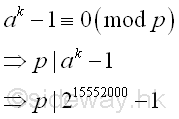###### Greatest Common Divisor

Since ak-1 is a very large number, before finding the greatest common divisor of n and ak-1,  ak-1 can be raised to the high power modulo n. Imply

Using squarings modulo

base  number; a=2; k=15552000; n=533
ak base 10 215552000
ai base 10 21  = 21 ก 2 (mod 533)
22 = 22 ≡ 4 (mod 533)
24 = 42 ≡ 16 (mod 533)
28 = 162 ≡ 256 (mod 533)
216 = 2562 ≡ 510 (mod 533)
232 = 5102 ≡ 529 (mod 533)
264 = 5292 ≡ 16 (mod 533)
2128 = 162 ≡ 256 (mod 533)
2256 = 2562 ≡ 510 (mod 533)
2512 = 5102 ≡ 529 (mod 533)
21024 = 5292 ≡ 16 (mod 533)
22048 = 162 ≡ 256 (mod 533)
24096 = 2562 ≡ 510 (mod 533)
28192 = 5102 ≡ 529 (mod 533)
216384 = 5292 ≡ 16 (mod 533)
232768 = 162 ≡ 256 (mod 533)
265536 = 2562 ≡ 510 (mod 533)
2131072 = 5102 ≡ 529 (mod 533)
2262144 = 5292 ≡ 16 (mod 533)
2524288 = 162 ≡ 256 (mod 533)
21048576 = 2562 ≡ 510 (mod 533)
22097152 = 5102 ≡ 529 (mod 533)
24194304 = 5292 ≡ 16 (mod 533)
28388608 = 162 ≡ 256 (mod 533)
216777216 = 5092 ≡ 285 (mod 533)
233554432 = 2852 ≡ 518 (mod 533)
267108864 = 5182 ≡ 190 (mod 533)
ak base 10 28388608+4194304+2097152+524288+262144+65536+16384+2048+1024+512
ak base 10 28388608*24194304*22097152*2524288*2262144* 265536*216384*22048*21024*2512
ak base 10 256*16*529*256*16*510*16*256*16*529 ≡ 1 (mod 533)

Imply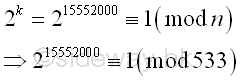The algorithm returns a fail response, because the number n divides ak-1 and n is the greatest common divisor of n and ak-1. Imply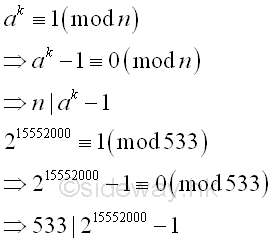Therefore every prime factor of number n divides ak-1, imply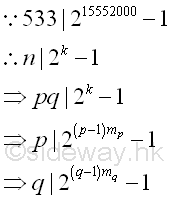Since k is a very large number, usually the algorithm fails because k is the multiple of the product of p-1 and q-1, imply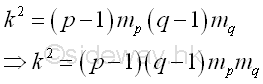One way is to select a smaller k so that k is not the common multiple of both p-1 and q-1. Let B=3, Imply

Integer B-smooth number Prime Factors number
k 3 29*35 = 512*243 124416

Therefore for B=3, k3 or (p3-1)m3 is equal to 124416.

###### Fermat's Little Theorem

let a=2, by Fermat's little theorem, let p be one of the prime factors of n, imply p divides ak-1.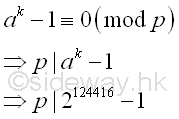###### Greatest Common Divisor

Since ak-1 is a very large number, before finding the greatest common divisor of n and ak-1,  ak-1 can be raised to the high power modulo n. Imply

Using squarings modulo

base  number; a=2; k=124416; n=533
ak base 10 2124416
ai base 10 21  = 21 ก 2 (mod 533)
22 = 22 ≡ 4 (mod 533)
24 = 42 ≡ 16 (mod 533)
28 = 162 ≡ 256 (mod 533)
216 = 2562 ≡ 510 (mod 533)
232 = 5102 ≡ 529 (mod 533)
264 = 5292 ≡ 16 (mod 533)
2128 = 162 ≡ 256 (mod 533)
2256 = 2562 ≡ 510 (mod 533)
2512 = 5102 ≡ 529 (mod 533)
21024 = 5292 ≡ 16 (mod 533)
22048 = 162 ≡ 256 (mod 533)
24096 = 2562 ≡ 510 (mod 533)
28192 = 5102 ≡ 529 (mod 533)
216384 = 5292 ≡ 16 (mod 533)
232768 = 162 ≡ 256 (mod 533)
265536 = 2562 ≡ 510 (mod 533)
ak base 10 265536+32768+16384+8192+1024+512
ak base 10 265536*232768*216384*28192*21024*2512
ak base 10 510*256*16*529*16*529 ≡ 469 (mod 533)

Imply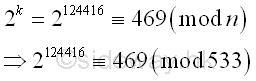The greatest common divisor of n and ak-1 is

Using Euclid's algorithm

ak-1 n
2124416-1 533
469-1 533
468 533-468=65
468-7*65=13 65
13 65-5*13=0
13 0

Imply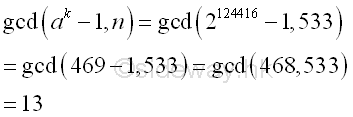Integer 13, the greatest common divisor of n and ak-1 is also the prime divisor of n. And p-1 is  3-smooth.

Integer B-smooth number Prime Factors number
p-1 3 22*31 12
k 3 29*35 = 512*243 124416
k/(p-1)   27*34 10368

Besides, for B=5

Integer B-smooth number Prime Factors number
p-1 3,5 22*31 12
q-1 5 23*51 40
k5 5 29*35*53 = 512*243*125 15552000
k5/(p-1)(q-1)   24*34*52 32400

©sideway

ID: 120500008 Last Updated: 2012/5/16 Revision:Home (5)

Business

Management

HBR (3)

Information

Recreation

Hobbies (7)

Culture

Chinese (1097)

English (336)

Reference (66)

Computer

Hardware (149)

Software

Application (187)

Digitization (24)

Numeric (19)

Programming

Web (648)CSS (SC)

ASP.NET (SC)

HTML

Knowledge Base

Common Color (SC)

Html 401 Special (SC)

OS (389)

MS Windows

Windows10 (SC)

.NET Framework (SC)

DeskTop (7)

Knowledge

Mathematics

Formulas (8)

Number Theory (206)

Algebra (20)

Trigonometry (18)

Geometry (18)

Calculus (67)

Complex Analysis (21)

Engineering

Tables (8)

Mechanical

Mechanics (1)

Rigid Bodies

Statics (92)

Dynamics (37)

Fluid (5)

Control

Acoustics (19)

Biology (1)

Geography (1)

Copyright © 2000-2019 Sideway . All rights reserved Disclaimers last modified on 10 Feb 2019